GCSE Maths Ratio and Proportion

# Ratio and Proportion

Here you will find 20 GCSE ratio and proportion revision resources. As for each GCSE maths revision topic, we've included step by step guides, detailed examples, practice questions, exam questions and ratio and proportion worksheets! All GCSE questions are based on actual Edexcel, AQA and OCR exam questions with mark scheme and model answers provided.

Filter by:

Speed Distance Time

Proportion

Inverse Proportion

Compound Measures

Ratio

Rates of Change

Exchange Rates

Scale

Flow Rate

Conversion Graphs

Units of Measurement

Show all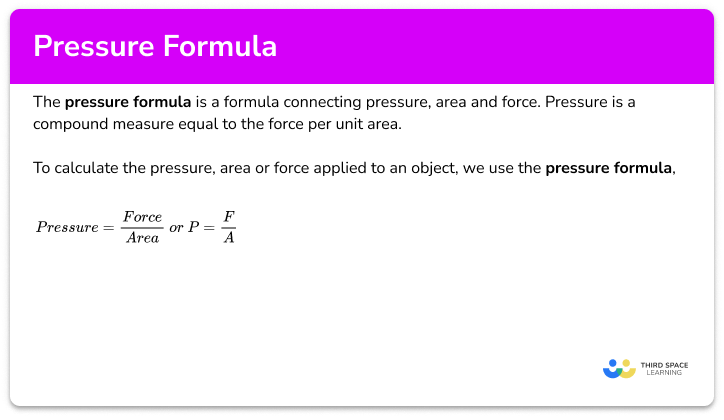Pressure formula

Step by step guide, detailed examples, practice questions, exam questions and pressure formula worksheets.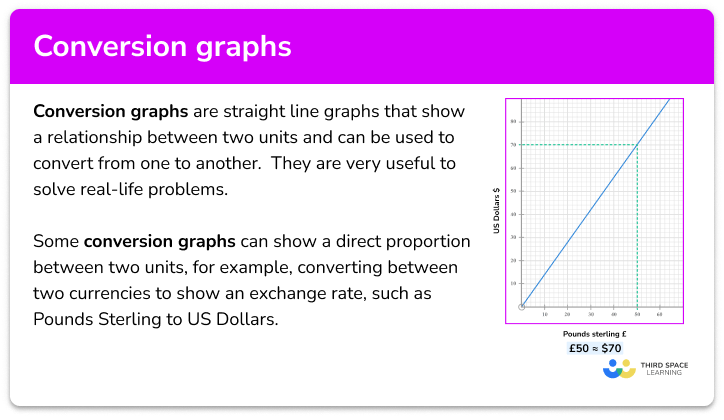Conversion graphs

Step by step guide, detailed examples, practice questions, exam questions and conversion graphs worksheets.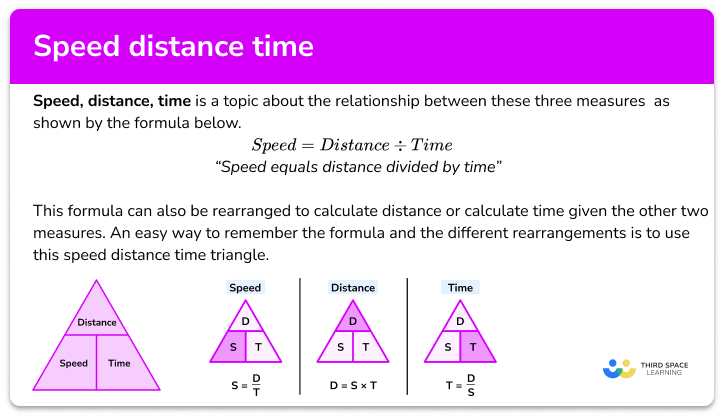Speed distance time

Step by step guide, detailed examples, practice questions, exam questions and speed distance time triangle worksheets.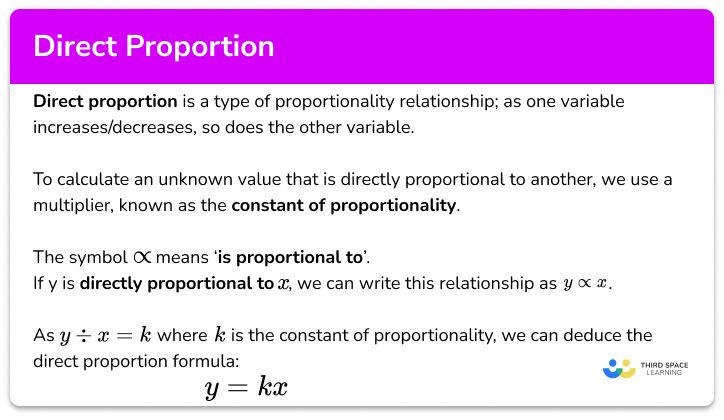Direct proportion

Step by step guide, detailed examples, practice questions, exam questions and direct proportion worksheets.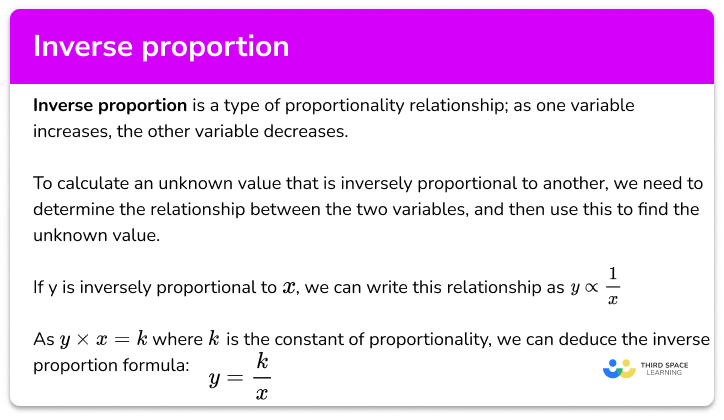Inverse proportion

Step by step guide, detailed examples, practice questions, exam questions and inverse proportion worksheets.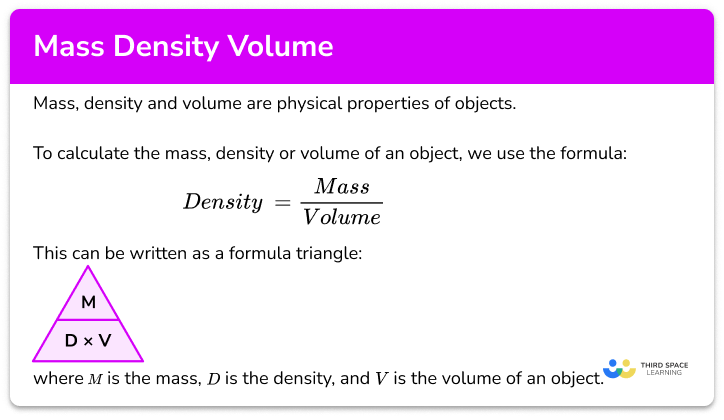Mass density volume

Step by step guide, detailed examples, practice questions, exam questions and mass density volume triangle worksheets.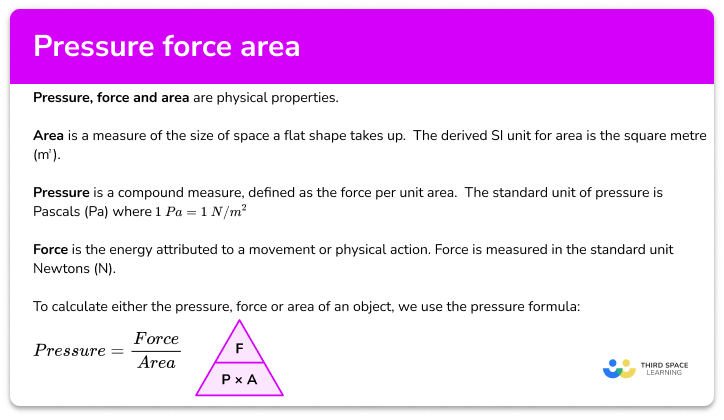Pressure force area

Step by step guide, detailed examples, practice questions, exam questions and pressure force area worksheets.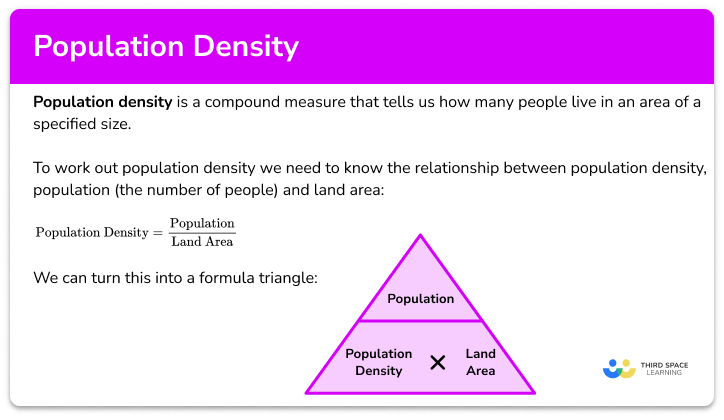Population density

Step by step guide, detailed examples, practice questions, exam questions and population density worksheets.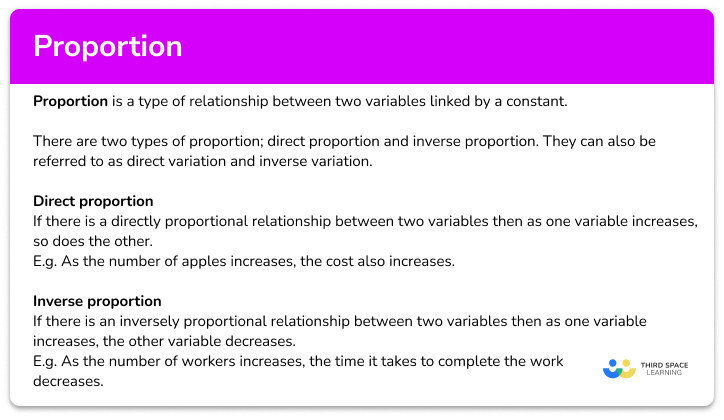Proportion

Step by step guide, detailed examples, practice questions, exam questions and proportion in maths worksheets.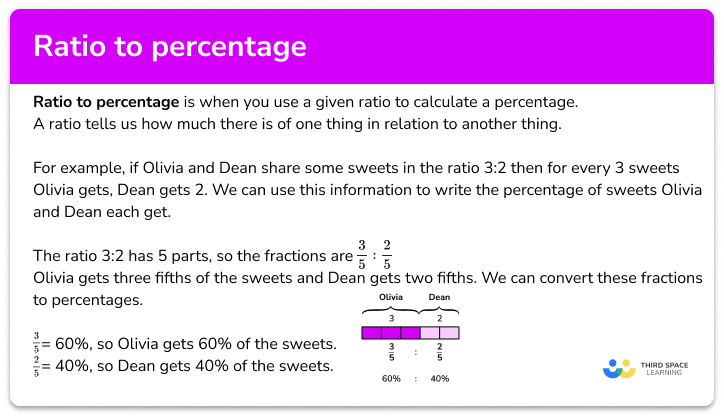Ratio to percentage

Step by step guide, detailed examples, practice questions, exam questions and ratio to percentage worksheets.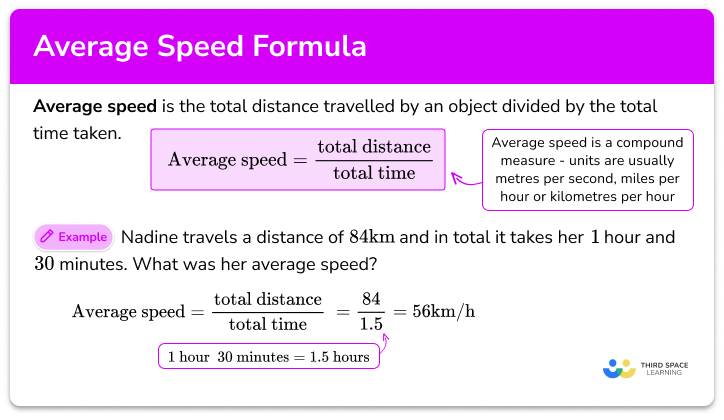Average speed formula

Step by step guide, detailed examples, practice questions, exam questions and average speed formula worksheets.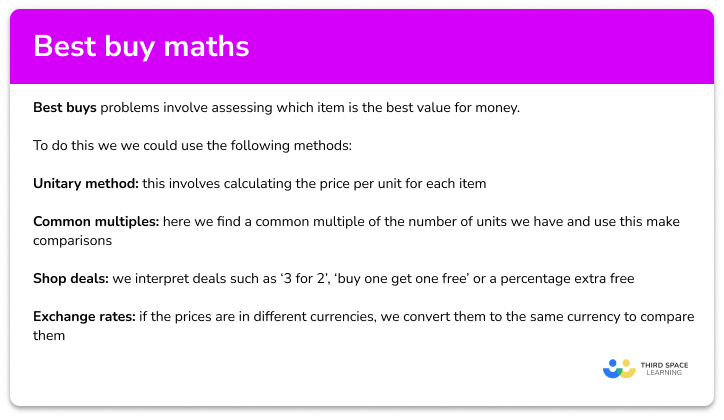Step by step guide, detailed examples, practice questions, exam questions and best buy maths worksheets.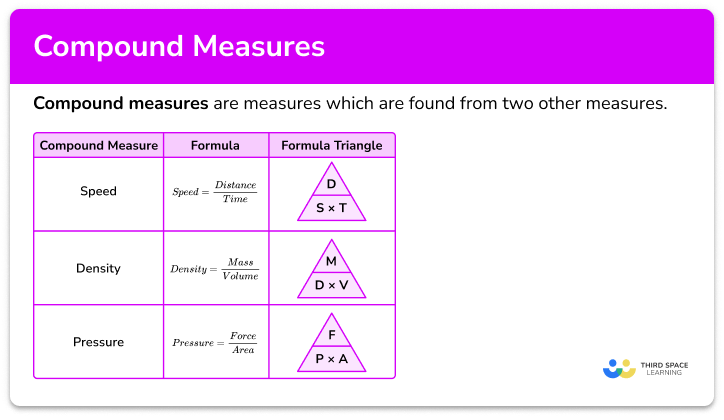Compound measures

Step by step guide, detailed examples, practice questions, exam questions and compound measures worksheets.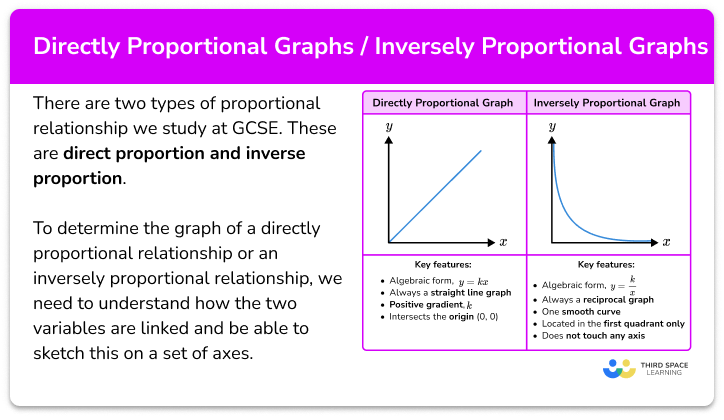Directly proportional graph/ inversely proportional graph

Step by step guide, detailed examples, practice questions, exam questions and directly proportional graph worksheets.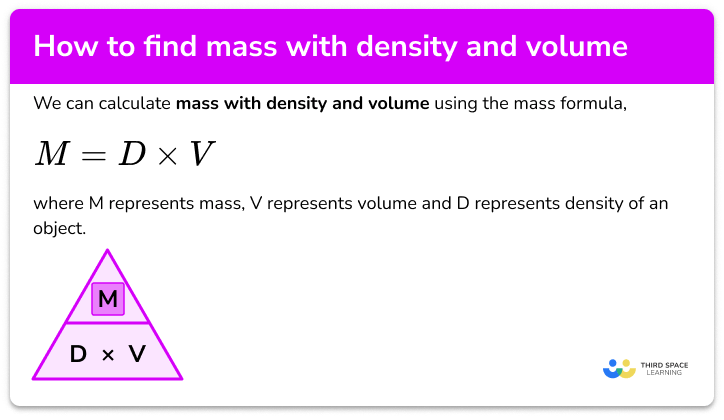How to find mass with density and volume

Step by step guide, detailed examples, practice questions, exam questions and how to find mass with density and volume worksheets.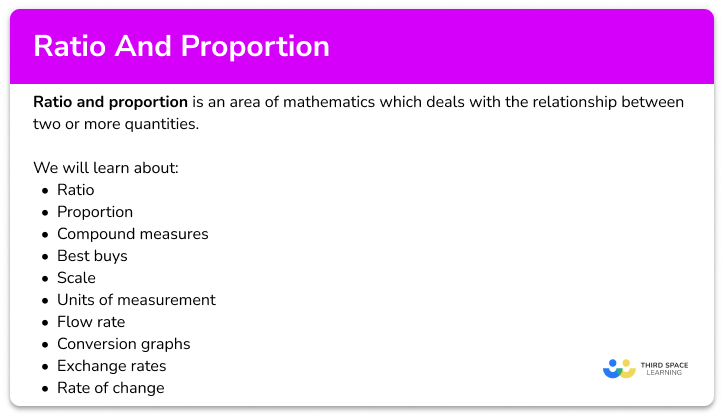Ratio and proportion – Maths GCSE

Step by step guide, detailed examples, practice questions, exam questions and ratio and proportion worksheets.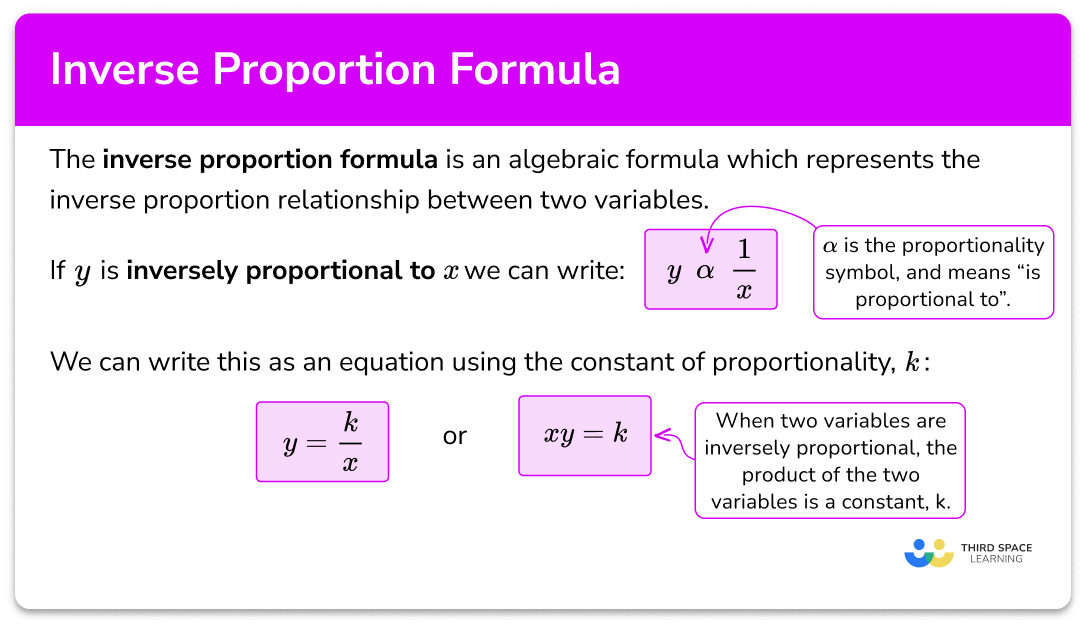Inverse proportion formula

Step by step guide, detailed examples, practice questions, exam questions and inverse proportion formula worksheets.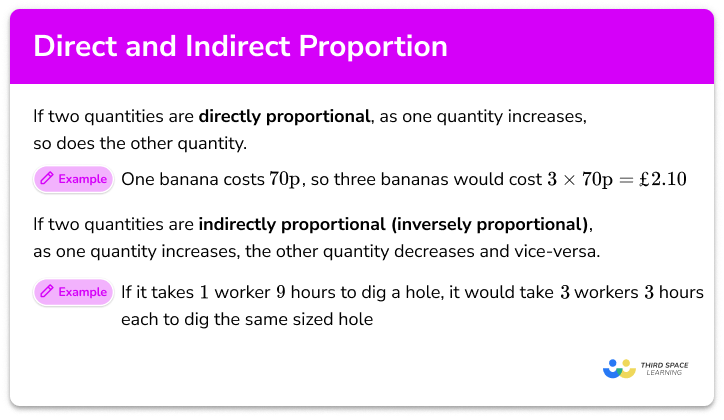Direct and indirect proportion

Step by step guide, detailed examples, practice questions, exam questions and direct and indirect proportion worksheets.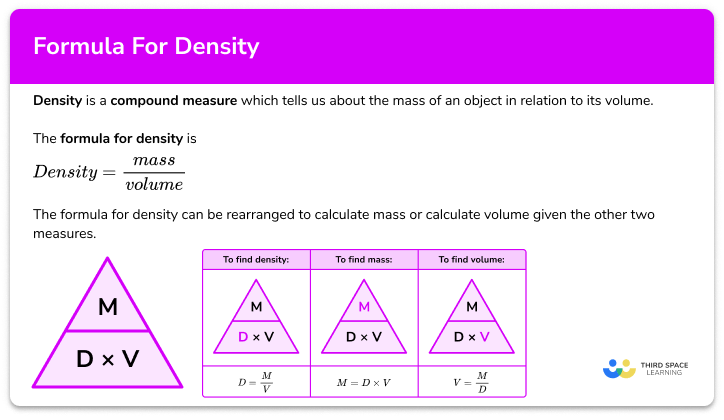Formula for density

Step by step guide, detailed examples, practice questions, exam questions and formula for density worksheets.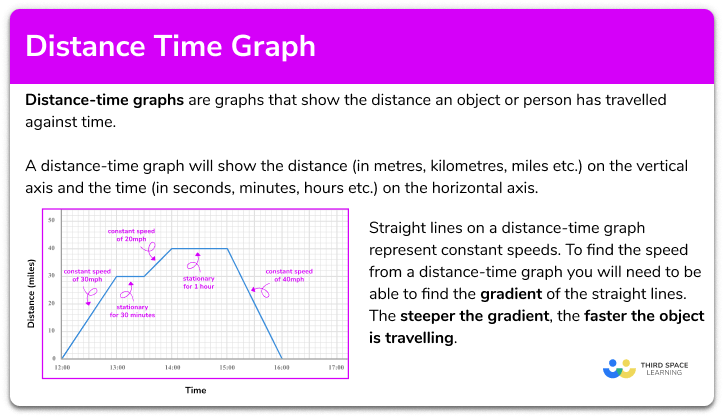Distance time graph

Step by step guide, detailed examples, practice questions, exam questions and distance time graph worksheets.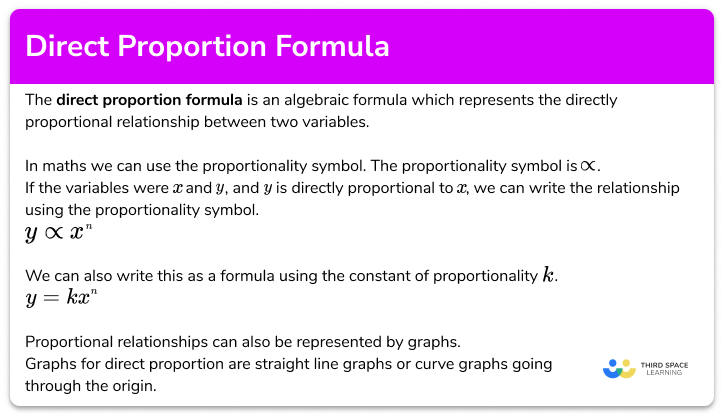Direct proportion formula

Step by step guide, detailed examples, practice questions, exam questions and direct proportion formula worksheets.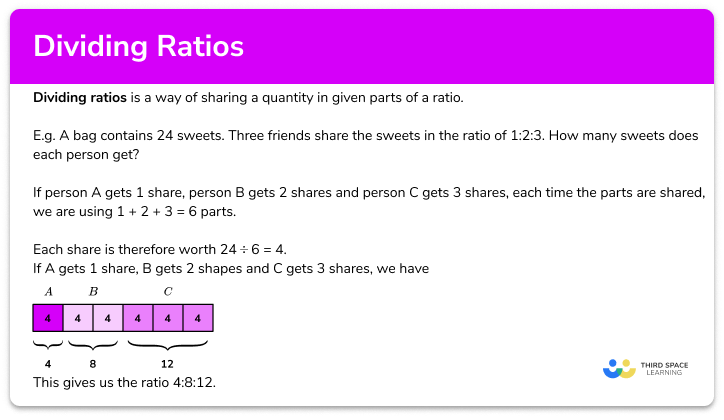Dividing ratios

Step by step guide, detailed examples, practice questions, exam questions and dividing ratios worksheets.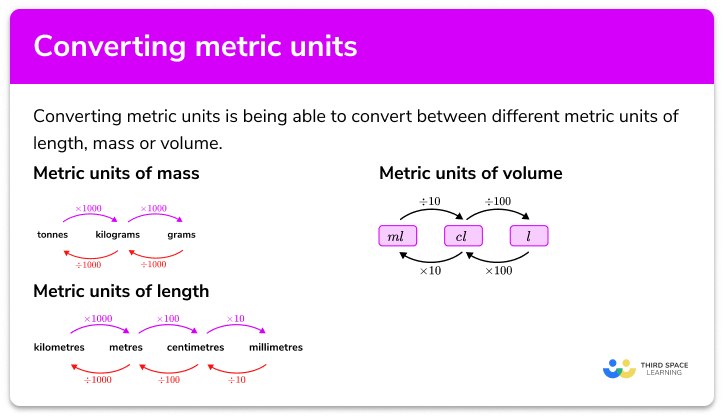Converting metric units

Step by step guide, detailed examples, practice questions, exam questions and converting metric units worksheets.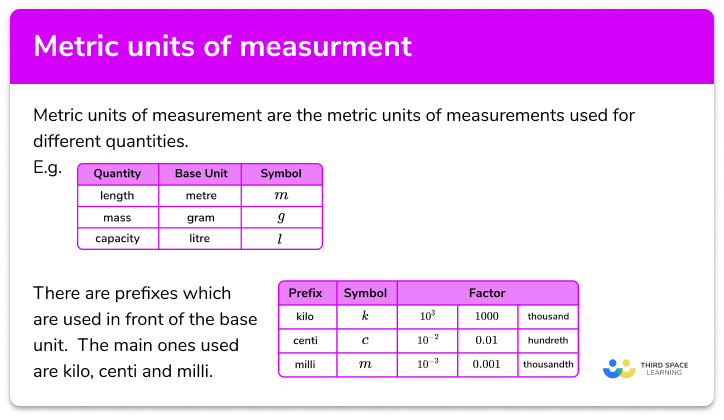Metric units of measurement

Step by step guide, detailed examples, practice questions, exam questions and metric units of measurement worksheets.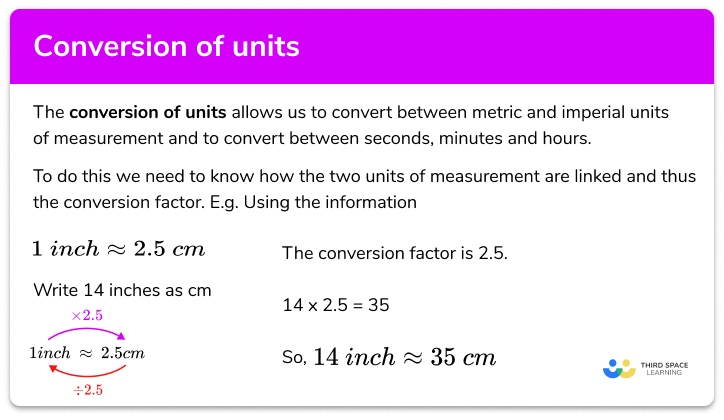Conversion of units

Step by step guide, detailed examples, practice questions, exam questions and conversion of units worksheets.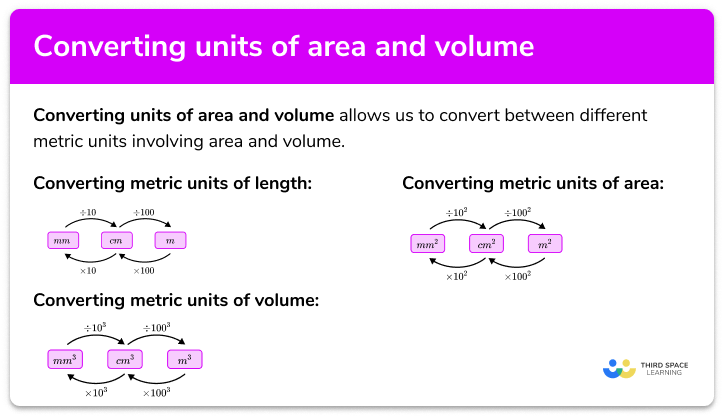Converting units of area and volume

Step by step guide, detailed examples, practice questions, exam questions and converting units of area worksheets.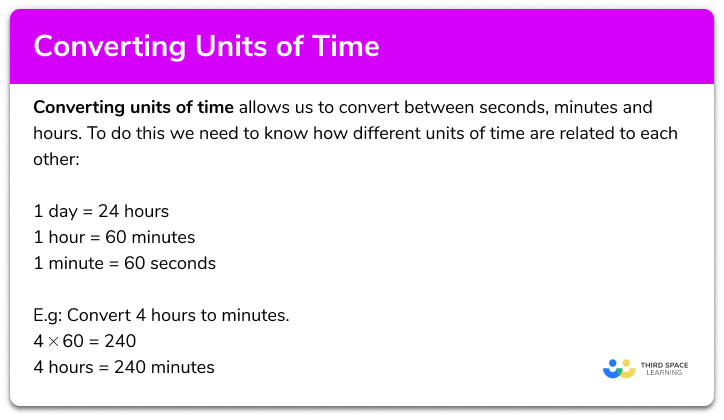Converting units of time

Step by step guide, detailed examples, practice questions, exam questions and converting units of time worksheets.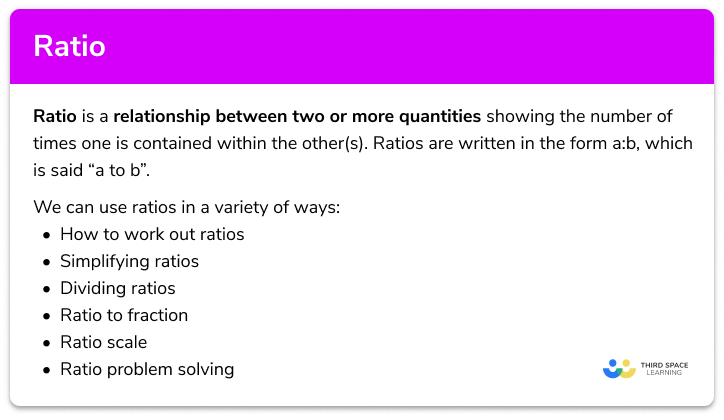Ratio

Step by step guide, detailed examples, practice questions, exam questions and ratio worksheets.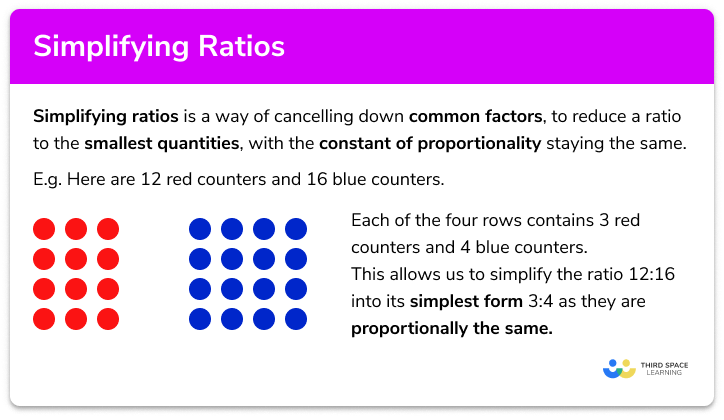Simplifying Ratios

Step by step guide, detailed examples, practice questions, exam questions and simplifying ratios worksheets.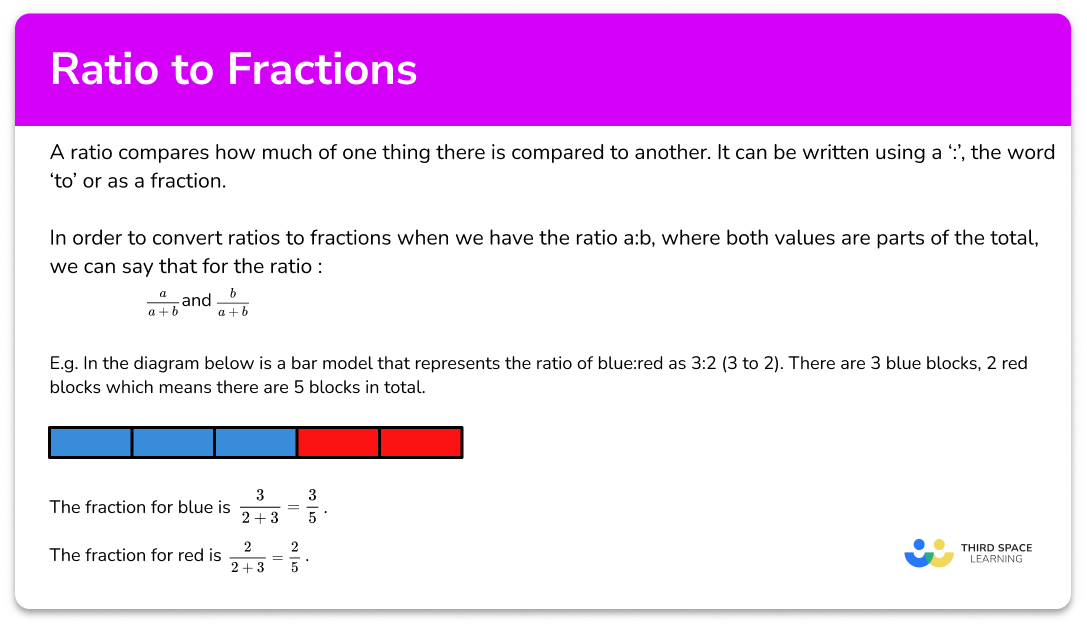Ratio to fraction

Step by step guide, detailed examples, practice questions, exam questions and ratio to fraction worksheets.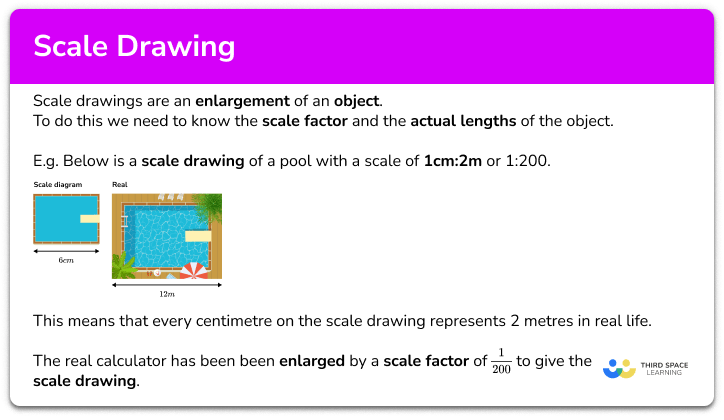Scale Drawing

Step by step guide, detailed examples, practice questions, exam questions and scale drawing worksheets.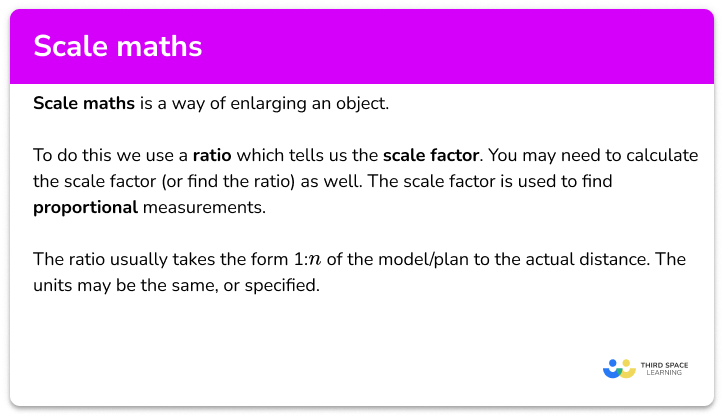Scale maths

Step by step guide, detailed examples, practice questions, exam questions and scale maths worksheets.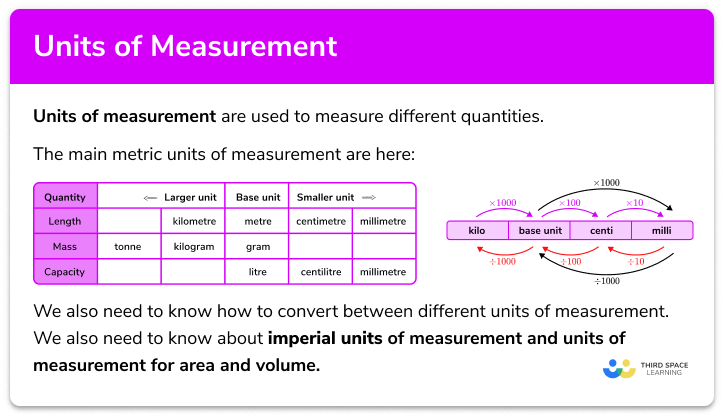Units of Measurement

Step by step guide, detailed examples, practice questions, exam questions and units of measurement worksheets.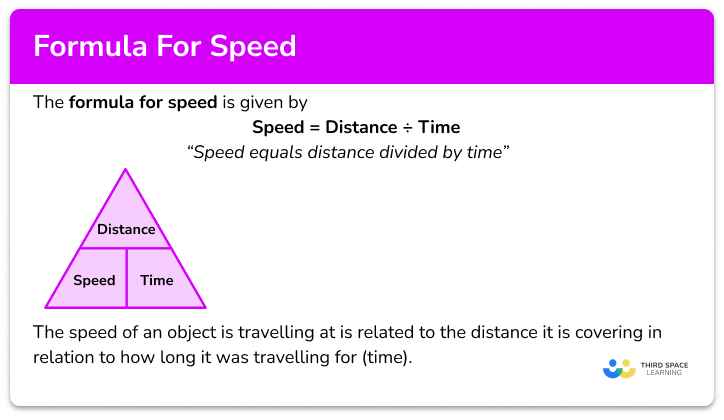Formula for speed

Step by step guide, detailed examples, practice questions, exam questions and formula for speed worksheets.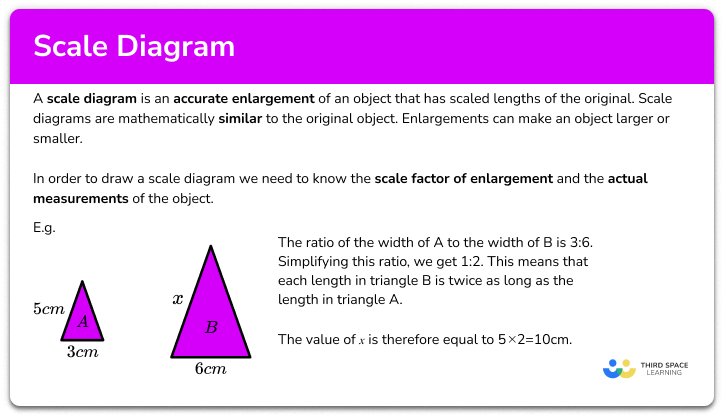Scale Diagram

Step by step guide, detailed examples, practice questions, exam questions and scale diagram worksheets.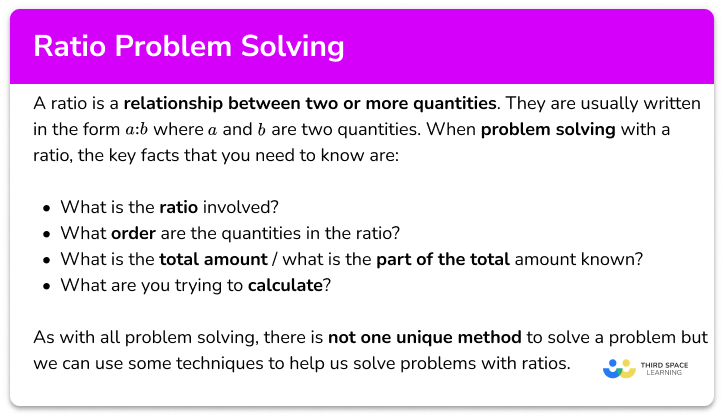Ratio problem solving

Step by step guide, detailed examples, practice questions, exam questions and ratio problem solving worksheets.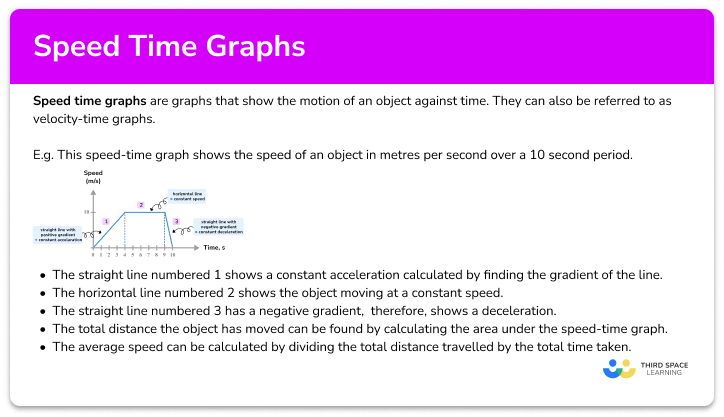Speed time graph

Step by step guide, detailed examples, practice questions, exam questions and speed time graph worksheets.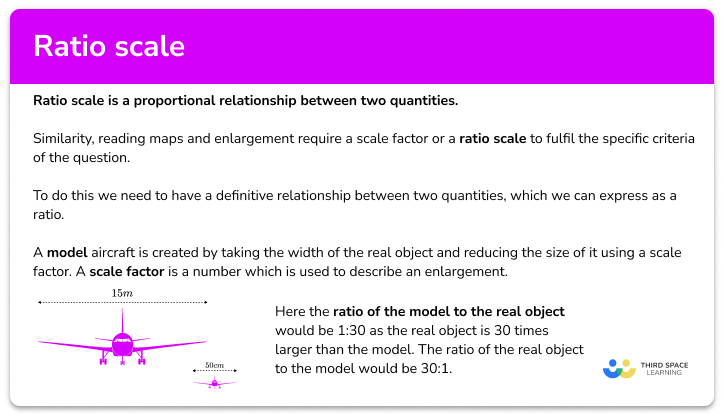Ratio scale

Step by step guide, detailed examples, practice questions, exam questions and ratio scale worksheets.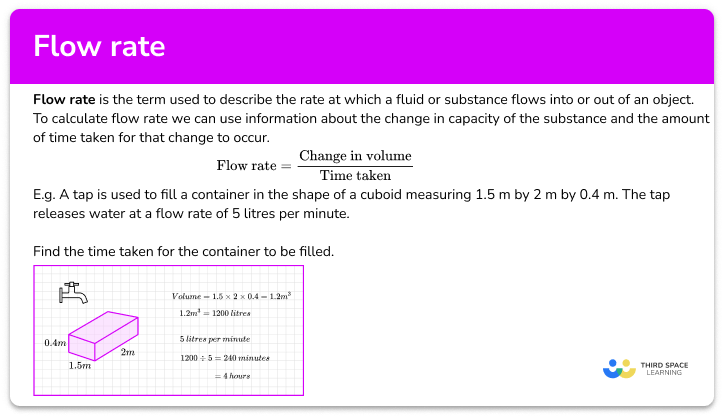Flow rate

Step by step guide, detailed examples, practice questions, exam questions and flow rate worksheets.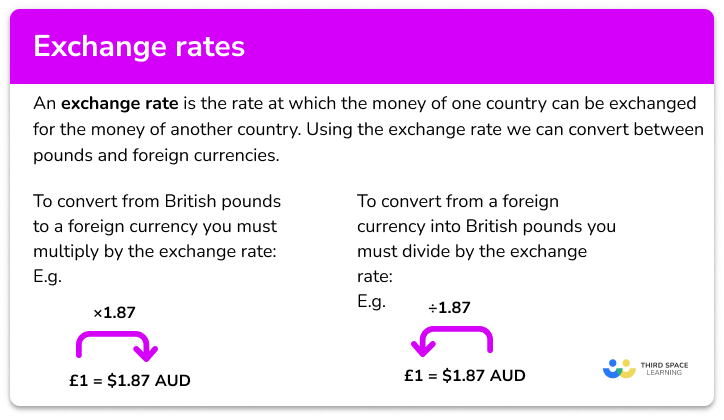Exchange rates

Step by step guide, detailed examples, practice questions, exam questions and how to work out exchange rates worksheets.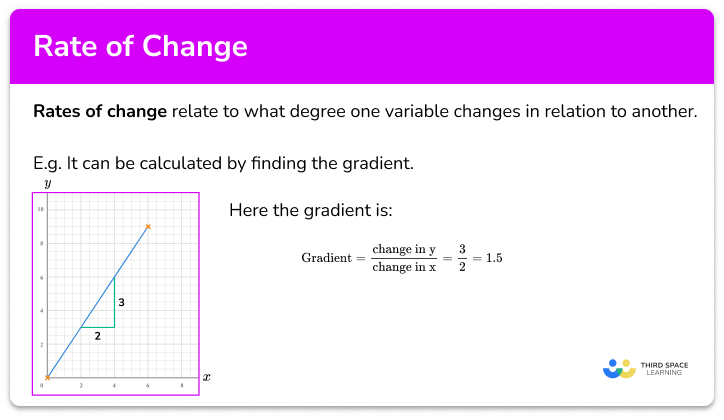Rate of change

Step by step guide, detailed examples, practice questions, exam questions and rate of change worksheets.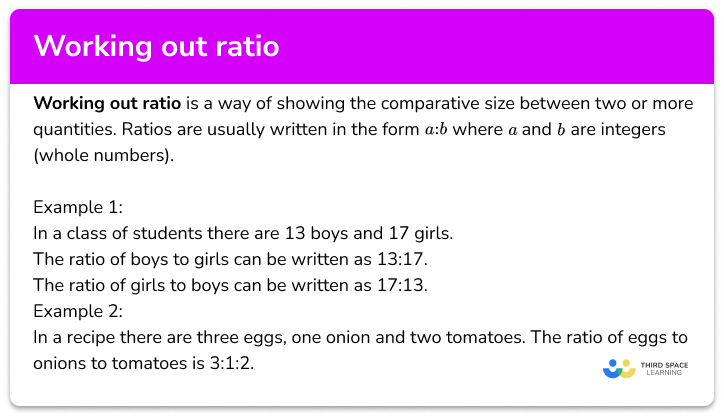How to work out ratio

Step by step guide, detailed examples, practice questions, exam questions and how to work out ratio worksheets.

One to one maths interventions built for KS4 success

Weekly online one to one GCSE maths revision lessons now available